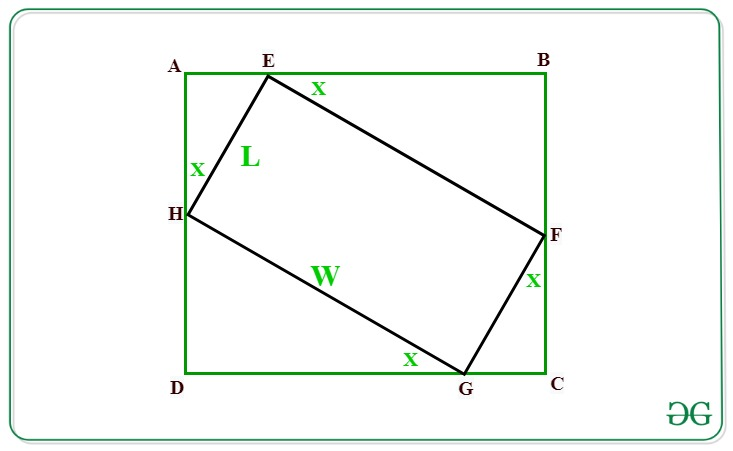# Maximum area of a Rectangle that can be circumscribed about a given Rectangle of size LxW

Given a rectangle of dimensions L and W. The task is to find the maximum area of a rectangle that can be circumscribed about a given rectangle with dimensions L and W.

Examples:

Input: L = 10, W = 10
Output: 200

Input: L = 18, W = 12
Output: 450

## Recommended: Please try your approach on {IDE} first, before moving on to the solution.

Approach: Let below is the given rectangle EFGH of dimensions L and W. We have to find the area of rectangle ABCD which is circumscribing rectangle EFGH.In the above figure:
Ifthenas GCF is right angled triangle.
Therefore,=>=>Similarly,Now, The area of rectangle ABCD is given by:

Area = (AE + EB)*(AH + HD) …..(1)

According to the projection rule:
AE = L*sin(X)
EB = W*cos(X)
AH = L*cos(X)
HD = W*sin(X)

Substituting the value of the above projections in equation (1) we have:Now to maximize the area, the value of sin(2X) must be maximum i.e., 1.
Therefore after substituting sin(2X) as 1 we have,Below is the implementation of the above approach:

## C++

 // C++ program for the above approach   #include    using namespace std;      // Function to find area of rectangle   // inscribed another rectangle of   // length L and width W   double AreaofRectangle(int L, int W)  {             // Area of rectangle       double area = (W + L) * (W + L) / 2;             // Return the area       return area;  }     // Driver Code   int main()   {              // Given dimensions       int L = 18;      int W = 12;             // Function call       cout << AreaofRectangle(L, W);      return 0;   }      // This code is contributed by Princi Singh

## Java

 // Java program for the above approach   import java.io.*;  import java.util.*;      class GFG{         // Function to find area of rectangle   // inscribed another rectangle of   // length L and width W   static double AreaofRectangle(int L, int W)  {             // Area of rectangle       double area = (W + L) * (W + L) / 2;             // Return the area       return area;  }         // Driver Code   public static void main(String args[])  {              // Given dimensions       int L = 18;      int W = 12;             // Function call       System.out.println(AreaofRectangle(L, W));  }   }      // This code is contributed by offbeat

## Python3

 # Python3 program for the above approach     # Function to find area of rectangle   # inscribed another rectangle of   # length L and width W  def AreaofRectangle(L, W):         # Area of rectangle    area =(W + L)*(W + L)/2    # Return the area    return area     # Driver Code  if __name__ == "__main__":       # Given Dimensions    L = 18   W = 12      # Function Call    print(AreaofRectangle(L, W))

## C#

 // C# program for the above approach   using System;     class GFG{         // Function to find area of rectangle   // inscribed another rectangle of   // length L and width W   static double AreaofRectangle(int L, int W)  {             // Area of rectangle       double area = (W + L) * (W + L) / 2;             // Return the area       return area;  }         // Driver Code   public static void Main(String []args)  {              // Given dimensions       int L = 18;      int W = 12;             // Function call       Console.Write(AreaofRectangle(L, W));  }   }      // This code is contributed by shivanisinghss2110

Output:

450.0


Time Complexity: O(1)
Auxiliary Space: O(1)

Attention reader! Don’t stop learning now. Get hold of all the important DSA concepts with the DSA Self Paced Course at a student-friendly price and become industry ready.

My Personal Notes arrow_drop_up